# How to divide fractions by whole numbers

Want to find out how to divide fractions by whole numbers? We've provided a step-by-step guide of how to divide fractions by whole numbers to teach you everything you need to know.## Step 1: Express teh whole number as a fraction

Express the whole number as a fraction by writing 1 as its denominator. If we want to divide 4/5 by 20, we first express 20 as a fraction. (Note: If given fraction is a mixed fraction, change it to improper fraction too)

## Step 2: Convert to multiplication

Change the division symbol to a multiplication symbol.

## Step 3: Rewrite the divisor as its reciprocal

We can do this by the switching the values of the numerator and denominator

## Step 4: Cancel common factors shared and simplify

Keep in mind that we can only cancel common factors if one value is a numerator and the other a denominator.

## Examples of how to divide fractions by whole numbers

Q1) What would be the result if we divide 3 (3 )/11 by 12?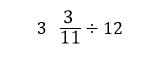Since we’re working with a mixed fraction, we change 3 (3 )/11 to an improper fraction. We then express 12 as a fraction : 12/1.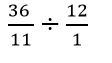Change ÷ to × then rewrite the divisor as its reciprocal.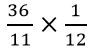Simplify and find the product of the expression.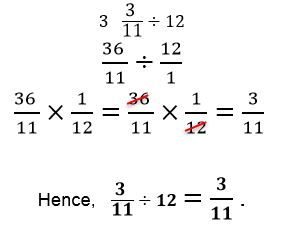Q2) A rainbow coloured string that is 3/4 yard long is to be divided into 15 smaller strips. How long is one strip of rainbow string?

To find the length of one strip, we divide the length of the string by the number of strips.

(3/4)÷15

We perform similar steps: express 15 as a fraction = 15/1.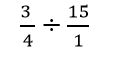Change ÷ to × then rewrite the divisor as its reciprocal.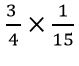Cancel out common factors and simplify.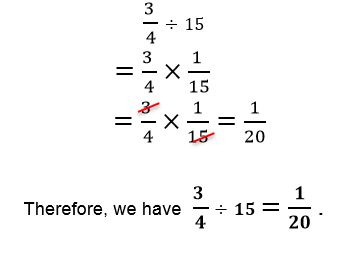#### Join today

The fastest way to practice

Unlock our complete testing platform and improve faster that ever.